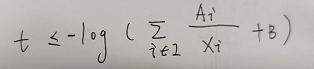# How to express log(sum(xi)) in CVXwhere Ai and B are constant and positive; xi are variables;
the RHS is concave and I dont know how to express in CVX;

This is `log-sum-inv`. In essence, the same as Log( {convex} ) . Make modifications, such as having `log(B)` rather than 0 as 1st element in vector argument of log_sum_exp,. no H^2 term, and A_i in place of GAMMA.

Note: edited to correct `log(B)` to `log(1/B)`.
Note: reverted to original., i.e., log(B), not log(1/B)

thanks im trying
but i still have some problems

Hello ! Do you solve problems? I want to ask your some questions.